# How to find Inverse of Matrix?

3,508 Views Updated: 31 Aug 2017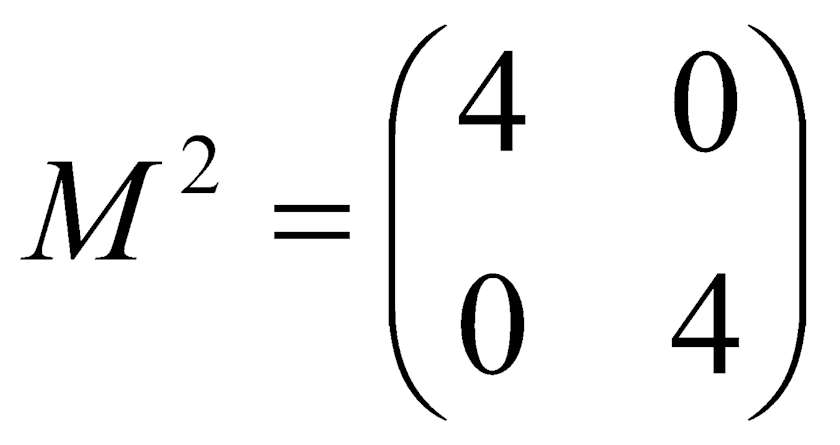Maths can be a troublesome thing for many, even those who enjoy solving problems and equations. Although, if you come to think of it, these problems strengthen the logical part of the mind and hence are really essential.

An important part of mathematical problem solving is finding the inverse of a matrix. In case you have been wondering how to calculate inverse matrix then here is a way to do it

How To Find Inverse Of Matrix

The use of inverse operations in algebra is to simplify problems that are generally too difficult to solve. The calculation of the inverse of a 3x3 matrix by hand is no means an easy job, but it is useful to know how it is done.

The first method is to create the adjugate matrix to find the inverse matrix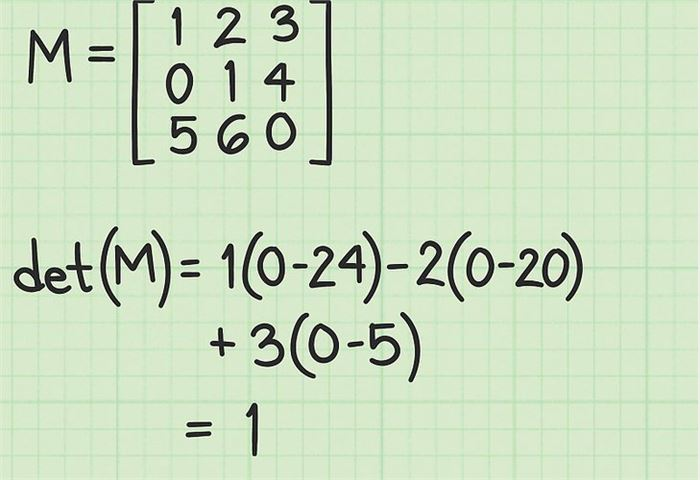(Image Courtesy: wikiHow)

#1. Finding the determinant of the matrix is the first step. If the determinant is 0 then no further calculation is required as the matrix has no inverse. In case of a 3x3 matrix, calculate the determinant by first.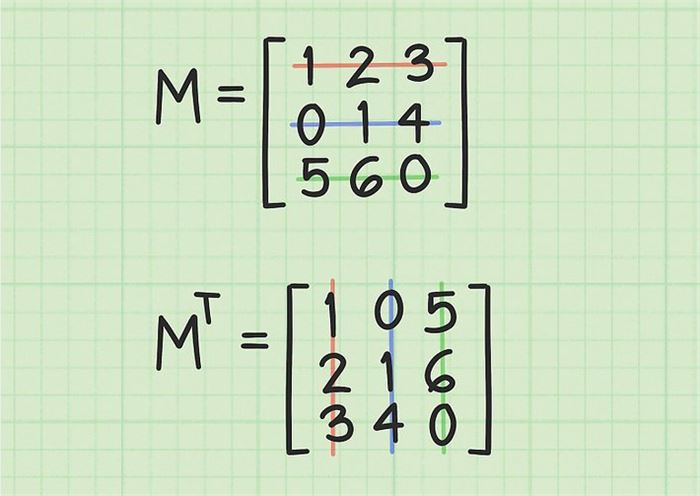(Image Courtesy: wikiHow)

#2. Transpose the original matrix which simply means that reflecting the matrix about the main diagonal, or uniformly, swapping the (i,j)th element and the (j,i)th. Transposing the terms of the matrix means that the main diagonal which is from the upper left to lower right is unchanged. The other way to deal transposing is by writing the first row as the first column, similarly the middle row as the middle column and the third row as the third column. If you notice the colored numbers in the diagram above, you will notice the changed positions.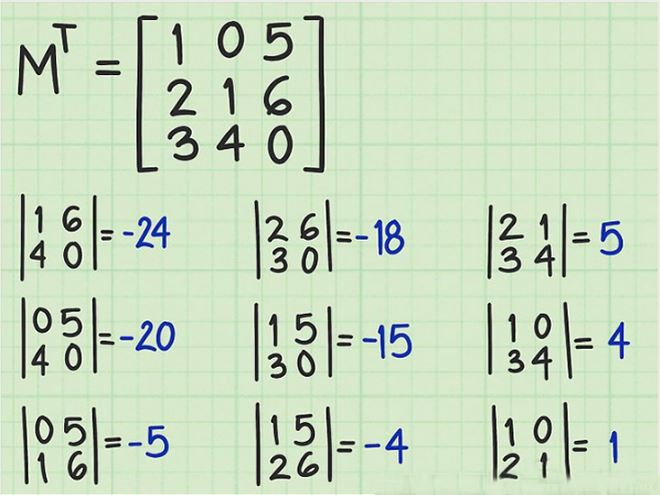(Image Courtesy: wikiHow)

#3. The third step is to find the determinant of each of the 2x2 minor matrices. There is an association between each of the newly transposed 3x3 matrices with its corresponding 2x2 “minor” matrix. In order to find the right minor matrix of each of the term, you need to highlight the row and column of the term you begin with. This will include five terms of the matrix. The four other terms that you are left with making up the minor matrix.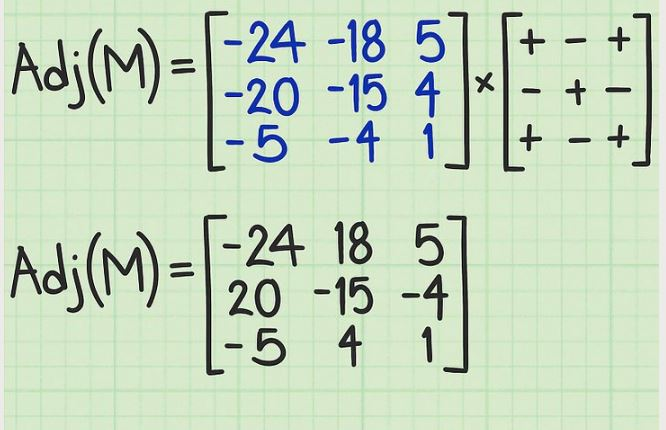(Image Courtesy: wikiHow)

#4. The fourth step is to create the matrix of cofactors. For this, you need to place whatever results you got from previous steps into a new matrix of cofactors which is done by aligning each matrix determinant with the corresponding position in the original matrix. After this, you have to reverse the sign of alternating terms of this new matrix, based on the “checkerboard” pattern which is given below.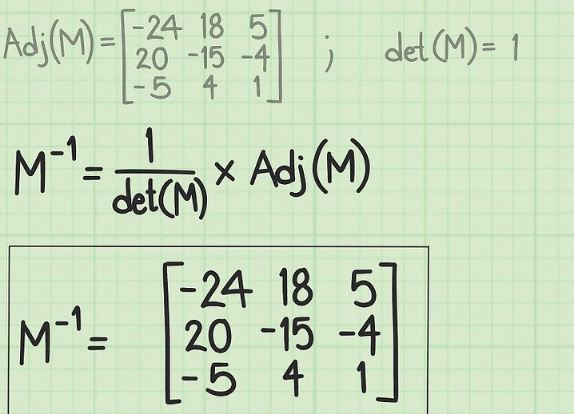(Image Courtesy: wikiHow)

#5. The last step is to divide each term of the adjugate matrix by the determinant. First of all, remember the determinant of M which was calculated right in the beginning to check if inverse was possible. Place the result of every single calculation into the spot of the original term. What you are left with is the inverse of the original matrix.

Have we made the calculations easier for you? Share your feedback using the comment box below.

(Featured Image Courtesy: Marked By Teachers)Posted by: Summer Posts: (3) Opinions: (11) Points: 365 Rank: 475

#### Related polls29 Opinions